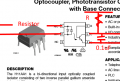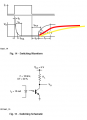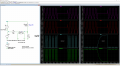# Can H11AA1 optocoupler detect 6V AC?

#### Ed. M.

Joined Nov 8, 2017
41
Hi,
I have several optocouplers I'm using to detect voltages in various wires. Most of the wires are run with 17VAC and I'm using input resistor values of 1.2-1.5KOhm, then run the output line 5 of the H11AA1 into my Arduino with the pins defined as INPUT_PULLUP. This seems to work fine.

I have another line that's only 6V. I'm thinking I need an input resistor of:
(6V-1.2V)/60ma = 80Ohm
The smallest resistor I have is 180Ohm so I'm trying to use that which should give me an input current of:
(6V-1.2V)/180Ohm = 27ma which should be enough to activate the optocoupler and give me an output current.

The odd thing is on my Arduino it's telling me the pin is going low (active), then high (off), then low, then high, etc. It just bounces around even though there's always current flowing through the input line.

Any idea why this could be happening? Would a true 80Ohm resistor fix it (if so, what wattage?) Thanks for your time I appreciate it.

#### Ed. M.

Joined Nov 8, 2017
41
I tried a 91Ohm resistor which should give me about 53ma input to the chip and I'm still getting the same behavior; the Arduino input bounces between low and high.

Here's what I don't understand. If I put more voltage through the line, it stabalizes at low. I'm doing the same thing with other lines that have 17VAC on them and I'm not seeing this behavior.

Last edited:

#### ronsimpson

Joined Oct 7, 2019
419
The data sheet said it takes 1.2V on the input before anything happens. (my words not theirs) I have used the H11AA1 to detect 120Vac/220vac. A 120V ac signal spends very little time below 1.2V. Now look at a 17vac signal. It spends much more time below 1.2V. A 6vac signal spends even more time where the current is too little. 6V is the average but part of the time the voltage is at 2V or 1V.

The H11AA1 is a little slow and probably the time for 120Vac to "cross zero" is so small the H11AA1 does not respond. Using a 6vac the current might be too low for 25% of the time. (made up that number) The H11AA1 certainly will see no current for a time. I think you need to put a 0.1uF cap across the output of the H11AA1. (input of the Arduino)

The RC on the output will cause the line to stay low longer and hold low for the zero crossing time.#### Ed. M.

Joined Nov 8, 2017
41
You're a genius! Even though I knew it was an AC line I completely forgot about how long it would spend below 1.2V. I had a 0.1uF cap lying around and that fixed it!

If I want to do the same with my 17VAC optos, would I use the same size capacitor? If not, how do you compute the size?

Thanks again.

#### RPLaJeunesse

Joined Jul 29, 2018
83
FYI most small LEDs don't want to see more than 20mA, so your 180 Ohm resistor is a little low. I'd think 20mA at the 6V peak of 8.5V might be even better, so that means using more than 364 Ohms. Try a 390 Ohm part and see how it goes. (Note: The H11AA1 is specified at a 10mA LED operating point.)

•Ed. M.

#### ronsimpson

Joined Oct 7, 2019
419
would I use the same size capacitor?
I did not bother to do the math.
If it works at 6V then it will also work at 17V.
If I really had to find the "right" cap. I would look output of the 6vac opto and watch the pulse up at zero crossing. Then add a capacitor until the pulse never reached 0.5V. But if you can live with 10mS delay or 100mS delay then error on the side of too large of a cap. I tried to draw a picture of capacitors delaying the rise time. The fall time will also slow but not as much.#### eetech00

Joined Jun 8, 2013
1,788
Hi,
I have several optocouplers I'm using to detect voltages in various wires. Most of the wires are run with 17VAC and I'm using input resistor values of 1.2-1.5KOhm, then run the output line 5 of the H11AA1 into my Arduino with the pins defined as INPUT_PULLUP. This seems to work fine.

I have another line that's only 6V. I'm thinking I need an input resistor of:
(6V-1.2V)/60ma = 80Ohm
The smallest resistor I have is 180Ohm so I'm trying to use that which should give me an input current of:
(6V-1.2V)/180Ohm = 27ma which should be enough to activate the optocoupler and give me an output current.

The odd thing is on my Arduino it's telling me the pin is going low (active), then high (off), then low, then high, etc. It just bounces around even though there's always current flowing through the input line.

Any idea why this could be happening? Would a true 80Ohm resistor fix it (if so, what wattage?) Thanks for your time I appreciate it.
Hi

The image below is a simple demonstration of what was happening with your circuit.

The schematic circuit with the capacitor is shown below. The simulation is configured to switch the input signal between 6vrms and 0, and will remove the input signal from 80ms to 140ms.

The graph representing the circuit without the capacitor is the one on the left (marked WO/CAP).
You can see the voltage switching between 0 and 5v in sync with the sinewave. Your adruino was correct.The graph representing the circuit with the capacitor is the one on the right (marked W/CAP).
This one has a capacitor that helps smooth the sinewave signal so that the output voltage remains relatively constant.

eT#### RPLaJeunesse

Joined Jul 29, 2018
83
Thanks for the confirmation that 390 Ohms should work just fine.